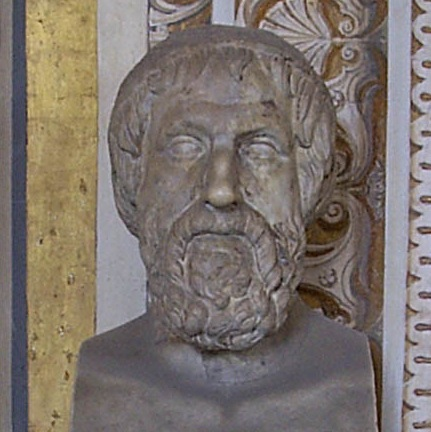Figurate numbers are numbers that arise from the natural sequence of particular geometrical arrangements of points, and have been studied at least since the time of Pythagoras (570-495 BC).

For example. the fourth triangular number, which corresponds to a triangular arrangement of ten points like sois called the tetractys in Greek, and was an important symbol in the Pythagorean religion. (Pythagoras and his followers had a very mystical view of mathematics, believing numbers reflected reality's deepest truths.)

Figurate numbers were subsquently studied by many important medieval and later mathematicians, most notably Euler (1707-1783).

Using the app below, investigate the properties of figurate numbers and see what interesting relationships you can uncover.

Figurate Numbers
Visualising relationships between shapes and numbers